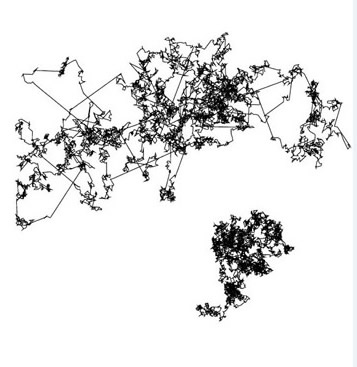May 22 - 31, 2013## Short Course on Fractional PDEs

Location: Room 110, 182 George Street

Time: 12:00-1:00pm

Lecture 1

22 May, 2013, Lecturer: Prof. Fawang Liu
Topic: Numerical methods and analysis of the time, space and time-space fractional PDEs

Lecture 2

23 May, 2013, Lecturer: Prof. Zhi-zhong Sun
Topic: Finite difference methods for the fractional sub-diffusion equation

PDF

Lecture 3

24 May, 2013, Lecturer: Prof. Fawang Liu
Topic: Numerical methods for variable order fractional partial differential equations

PDF

Lecture 4

29 May, 2013, Lecturer: Prof. Fawang Liu
Topic: Numerical and analytical solutions for the multi-term time-space fractional PDEs

PDF

Lecture 5

30 May, 2013, Lecturer: Prof. Zhi-zhong Sun
Topic: Finite difference methods for the fractional diffusion-wave equation

Lecture 6

31 May, 2013, Lecturer: Prof. Fawang Liu
Topic: Analysis of implicit numerical methods for the space-time fractional Bloch-Torrey equations

PDF

Prof. Fawang Liu
Professor, School of Mathematical Science
Queensland University of Technology, Australia
Research Interests: Numerical analysis; Fractional Differential Equations; Numerical methods of singular perturbation, adsorbate transport and microwave heating problems and saltwater
intrusion into heterogeneous aquifer systems.

Prof. Zhi-zhong Sun
Professor, Department of Mathematics
Southeast University, P. R. China
Research Interests: Numerical solutions for nonlinear PDEs; Numerical analysis; Error estimates of numerical solutions for fractional PDEs; Numerical solutions for Schrodinger equations, Cahn-Hilliard Equations.

For the latest developments of Fractional PDEs, please follow the upcoming symposium:
International Symposium on Fractional PDEs: Theory, Numerics and Applications, June 3 - 5, 2013.